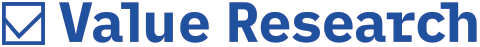# Two faces of the Sensex

##### Why the price-to-earnings and the price-to-book-value ratios of the Sensex tell different stories about its valuations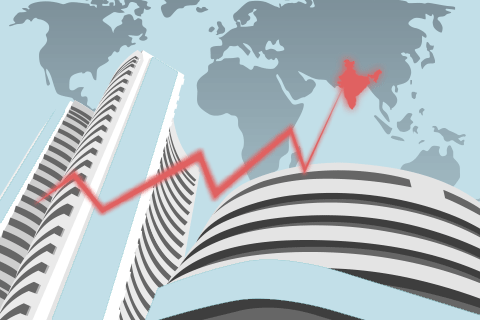In India, the S&P BSE Sensex index is frequently used to gauge market valuations. Two popular valuation measures are the price to earnings (P/E) and price to book (P/B) ratios. When applied to the long-term Sensex data, they, interestingly, tell very different stories.

See Figure 1. The P/E chart suggests that the current valuation of the Sensex is way above the median level. Also, the current P/E level is more than what it was in March 2010.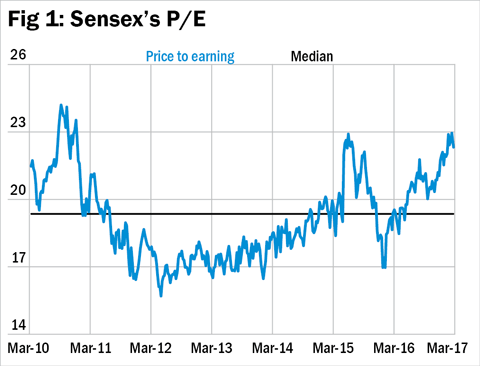When we look at the P/B chart (see Figure 2), it is still below the median level and much lower than what it was in March 2010. Thus, as per the P/E ratio, the market seems to be expensive, whereas as per the P/B ratio, the market seems to be undervalued in historical terms.

What could be the reason for this anomaly? If you thought that this is caused by a change in the Sensex's sectoral composition, our analysis suggests, that's not the case.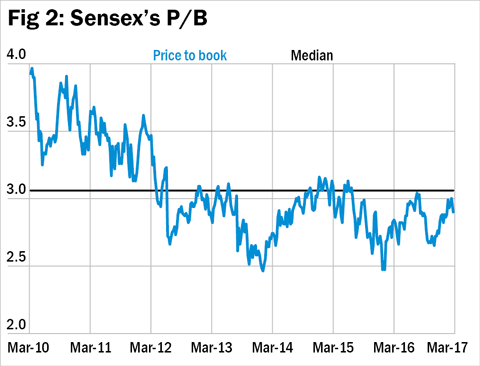To decode the mystery, let's first recall how the P/E and P/B ratios are calculated. In order to find the P/E ratio, price is divided by earnings per share. To calculate the P/B ratio, price is divided by book value (or net worth) per share. So if earnings remain muted, the P/E ratio will show an increase. But an increase in book value over time will dampen the P/B ratio.

On an aggregate basis, the book value of the Sensex companies has risen almost twice since March 2010, whereas the total earnings of the Sensex companies have risen by only one-and-a-half times. This means the EPS has not kept pace with book value.

Let's see this argument from an ROE perspective (see Figure 3). Return on equity (or return on net worth), which is calculated as net profit divided by book value, measures the efficiency of earnings. The RoE of the Sensex (based on market-cap weighted calculations) in March 2010 was 20 per cent. It has declined to 14.9 per cent in March 2017. This signals a fall in efficiency. Since less money is made on the equity base of Sensex companies, therefore, the P/E has lagged behind the P/B.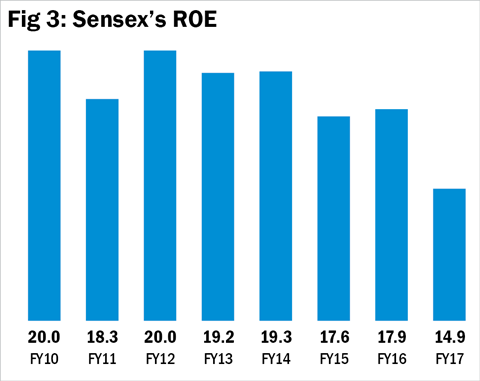The moral of the story is that while analysing stocks, you shouldn't rely on just one measure. Use a combination of tools. Relying on just one measure can be quite deceptive.